[BACK]
 Intelligent Automation & Soft ComputingDOI:10.32604/iasc.2021.013643Article

SDN Controller Allocation and Assignment Based on Multicriterion Chaotic Salp Swarm Algorithm

1Department of Information Technology, Sri Venkateswara College of Engineering, Sriperumbudur, Chennai, Tamilnadu, India
2Department of Electronics and Communication, Sri Venkateswara College of Engineering, Sriperumbudur, Chennai, Tamilnadu, India
*Corresponding Author: Suresh Krishnamoorthy. Email: ksuresh19871@gmail.com
Received: 30 August 2020; Accepted: 05 November 2020

Abstract: Increase in demand for multimedia and quality services requires 5G networks to resolve issues such as slicing, allocation, forwarding, and control using techniques such as software-defined networking (SDN) and network function virtualization. In this study, the optimum number of SDN multi-controllers are implemented based on a multi-criterion advanced genetic algorithm that takes into consideration three key parameters: Switch controller latency, hopcount, and link utilization. Preprocessing is the first step, in which delay, delay paths, hopcount, and hoppaths are computed as an information matrix (Infomat). Randomization is the second step, and consists of initially placing controllers randomly, followed by an analytical hierarchical process evaluation that considers switch controller latency as the primary objective in the assignment process. In the third and last step, crossover and mutation genetic functions are implemented for a local search process until the best placement is found (when the primary objective threshold is reached). A novel chaos theory is applied to the salp swarm algorithm (SSA) for enhancing the optimizer’s performance in assigning the switch controllers. The SSA is a dynamic optimal algorithm for switch controller connections. To enhance convergence rate and precision, chaotic maps extract random parameters based on Gaussian distribution for better control in reducing local optima. A logistic chaotic map is selected after analyzing its performance based on unimodal and multimodal optimization. Evaluations are carried out on this proposed algorithm for 12 sets of topologies with two categories: Fewer and more switches. Simulation results show the highest efficiency in a computation time of 25 ms with varying thresholds and 12 ms with varying upper boundary utilization when compared with the particle swarm optimization (PSO) meta-heuristic algorithm. The effects of varying latency thresholds and upper boundary utilization on two set of topologies inferred more requests serviced with fewer controllers deployed.

Keywords: Software defined networking (SDN); advanced genetic algorithm; novel chaos theory; salp swarm algorithm

1  Introduction

Software-defined networking (SDN) provides cost-effective dynamic communications between the control plane and the data plane. The data plane is responsible for all traffic forwarding operations while the control plane handles all traffic decisions in the network. This separation provides flexibility and control over changing network conditions.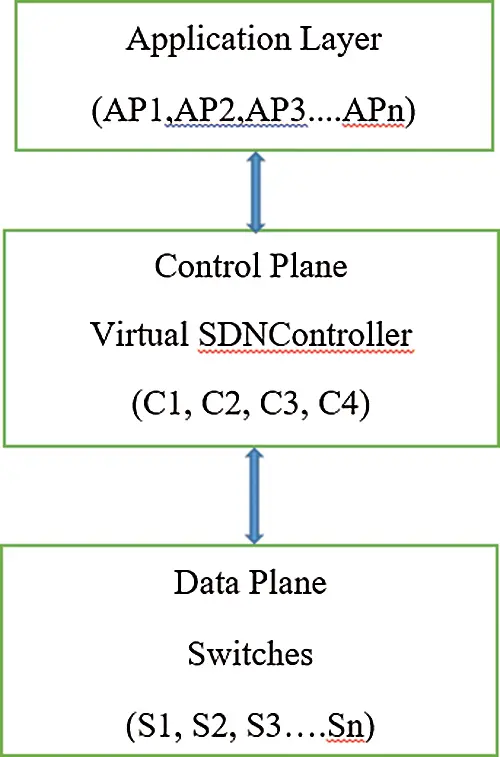Figure 1: SDN multi-controller general structure

In this study, the two proposed dynamic algorithms are

2.  Novel chaos theory on meta-heuristic algorithm.

The proposed advanced genetic algorithm is multi-criterion algorithm based on an analytic hierarchy process for optimum selection and placement of SDN controllers, in order to increase flexibility of network management decision-making. The novel chaotic salp swarm algorithm (NCSSA) is proposed for switch controller allocation in large-scale distributed SDN controllers. This algorithm applies novel chaotic maps to the salp swarm optimization algorithm (SSA) enhancing its performance in latency reduction between switches and controllers. To find the fitness function for the SSA, modeling of distributed multi-controllers is employed with C controllers (i = 1, 2, 3 … C) and S switches (j = 1, 2, 3 … S). Controllers receive service requests from switches and respond according to switching-forwarding rules. Each controller has a maximum limit of service up to N switches, as its resource usage is limited . Let us consider the mathematical model shown in (1) with controllers C = 7 and switches S = 10, with N matrix that defines the number of switches connected to controllers. Row matrix represents controllers and column matrix represents switches. The total number of ones in a row represents how many switches are connected to each controller. If the number of ones in each row exceeds the threshold value given by N matrix, the probability of failure increases.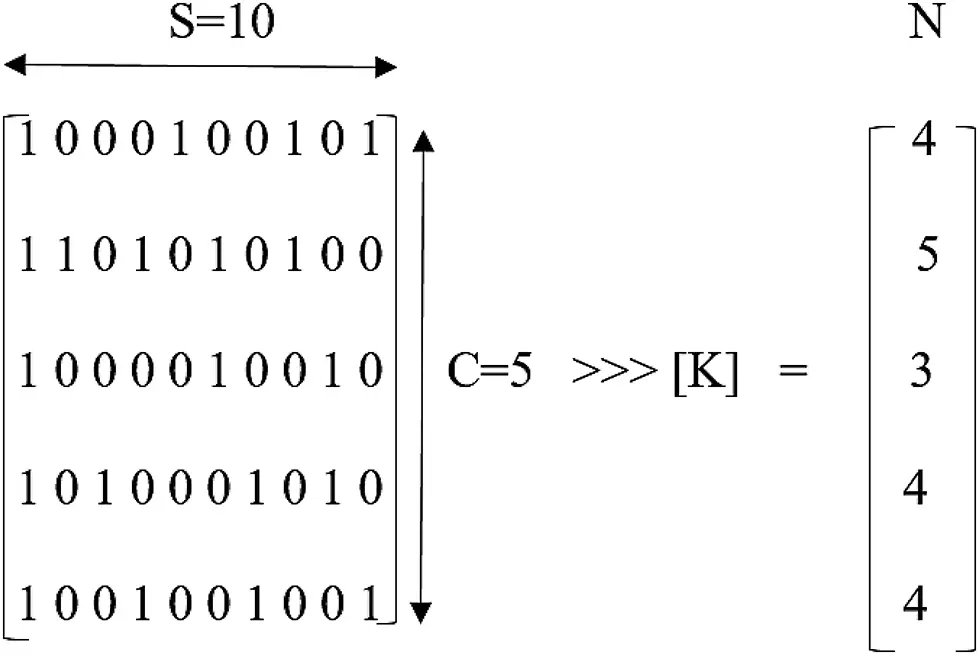The average response time (RTi) of controller (i = 1,2,3…. C) is calculated based on arrival rate (λi) and service rate (µi)where Ps is usage probability of system servers. The controller arrival rate (λi) is defined as summation of the arrival rate of all switches connected with their respective controllers.The average controller load (Loadi) can be calculated based on the number of requests received and serviced.where, u represents server utilization and s represents switches set.

The rest of study is organized as follows. Section 2 consists of a previous literature review. Section 3 describes the different criteria considered for analysis. Section 4 discusses the proposed controller placement algorithm. Sections 5 and 6 describe how chaotic maps are applied to the SSA for optimum switch controller allocation. Performance evaluation results are presented in Section 8. In Section 9, the authors offer their conclusions.

2  Related Works

In this section, the important literature on software-defined mobile network allocation is listed. A chaotic gray wolf optimizer controller framework is designed and evaluations are conducted on hierarchical switching topologies examining different quality of service (QoS) . An analytic hierarchy process technique is developed based on different criteria related to QoS. The efficiency with respect to link load balancing problem is analyzed . A review of 5G software and slicing techniques for service quality is undertaken, combining network slices and management in a single domain, and radio access network (RAN) virtualization and security challenges for 5G heterogenous systems are discussed . The study and development of capacity of controllers and link delays are discussed . A mathematical model for SDN controllers was designed that incurs low cost. This model determined the number and location of controllers, and the assignment of switches to controllers based on propagation delay. Rath  proposed a non-zero-sum algorithm for the selection and optimal placement of controllers in order to minimize deployment cost. This algorithm enhances optimization on each controller for dynamic network changes in real time. Gao  designed a framework for a controller placement algorithm. The PSO algorithm was developed based on swarm positions for controller placement. To enhance the optimizer’s performance, swarm with the best fitness function based on distance and latency was selected. Simulation results showed that the proposed algorithm performed better in terms of computation time and delay. However, this algorithm considers only a fixed number of deployed controllers and is not suitable for dynamic traffic load change. Bari  determined the requirement for the number of controllers and used integer linear programming to determine their positions. This study took into account criteria, such as a controller’s installation cost, the link cost between switches and controllers, and the linking of multi-controllers with each other. The inferences made from the above works and to what extent they were incorporated in the proposed algorithm are listed below.

•   The analytic hierarchy process is implemented in the advanced genetic algorithm for finding the best position of the controller.

•   The SDN multiple controller architecture is understood. How the data plane and control plane can be integrated in a single domain and the security challenges in a 5G heterogeneous system are discussed.

•   How controllers are deployed and assigned to switches based on propagation delay. This propagation delay is a metric considered for the proposed algorithm. Also considered are how to minimize latency due to propagation and processing, energy consumption, cost elements such as CAPEX and OPEX, and maximizing resilience and reliability.

•   Optimization of controllers based on a non-zero sum algorithm and integer linear programming is explained.

•   The design of the framework for optimizing the placement of a fixed number of controllers using the particle swarm optimization (PSO) algorithm.

Unlike previous articles, this article considers three different metrics for the optimum deployment of controllers and the optimal establishment of switch controller connections, based on the NCSSA.

3  Multicriterion Analysis

In this section, different criterion to be analyzed for controller placement and switch allocation to controller are discussed in detail [13,14].

3.1 Switch Controller Latency

Switch controller latency is most important parameter for controller placement. Increase in delay for switch servicing by controller will affect performance to the network activity.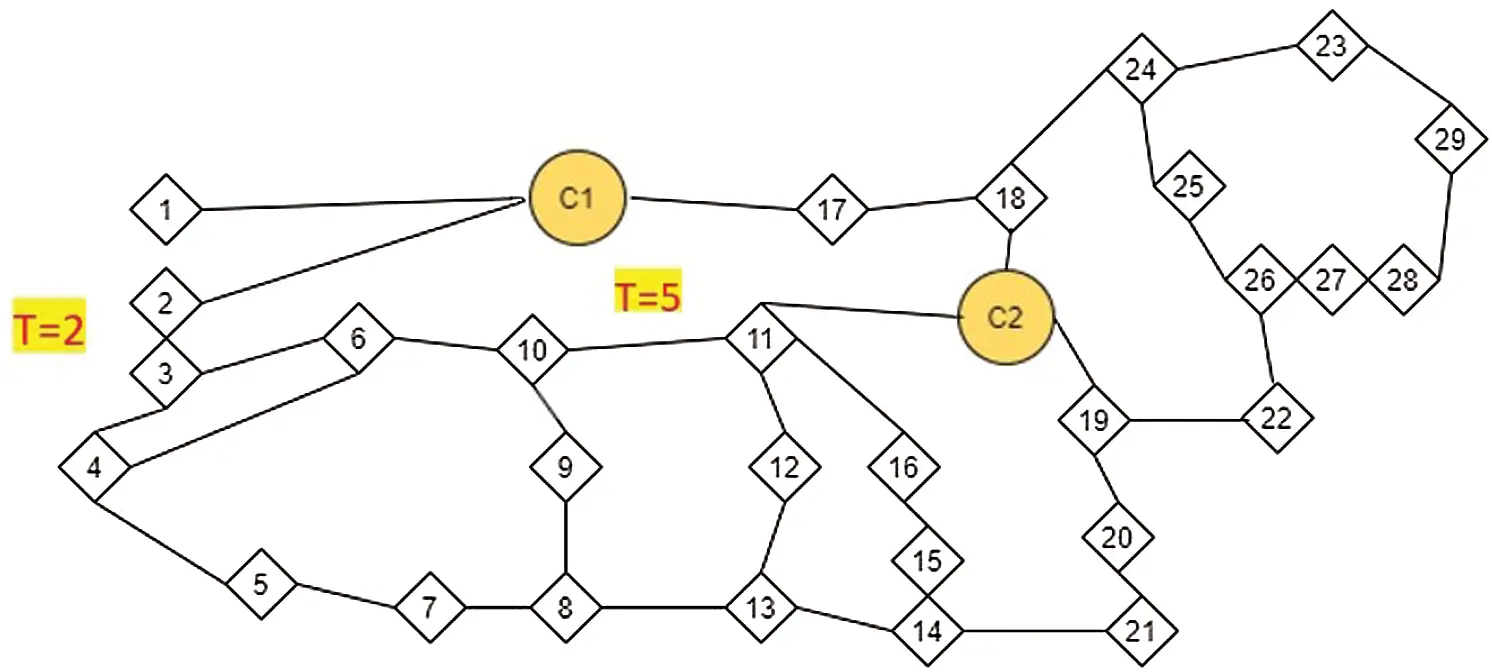Figure 2: Controller switch assignment

In Fig. 2 there are two controllers C 1 and C 2 load imbalance can be viewed, where majority nodes are connected to C 2 and minimum nodes are connected to C 1, for example, let us consider node 6 is assigned to C 2 through two nodes 10 and 11 rather assigned to C 1 through nodes 2 and 3. switches 10 and 11 are used by nodes 9, 8 and 12,13, respectively, to connect with C 2. Hence the usage of links through switch 10 and 11 will be considerably busy increasing delays and probability of failure .

3.2 Hop Count

Hop count is defined as no of nodes present as intermediate between source and destination. For every hop it incurs latency which is important criterion to be considered for allocating switches to controllers. It is not possible to allocate only based on hopcount, since other parameters like delay, utilization should also be considered. For example node 6 can be allocated to either C 1 or C 2 since both are having same hop count. On considering latency through nodes 10 and 11 the efficiency decreases when connected to C 2 rather when connected to C 1.

Link utilization criterion is defined as no of users currently using particular link indicates bandwidth utilization by traffic flow. Let us consider node 6 is connected to C 1 through the path 3 → 2 having transversal value (T) = 2 on particular link. In other case, if node 6 is connected to C 2 through the path 10 → 11 having T = 5 on particular link. Having more utilization bandwidth will definitely affect efficiency of the system and considering only one of above discussed parameters will not produce good result. Hence correct decision should be taken by administrators based on different preferences.

4  Controller Placement Algorithm

In order to find vicinity of controllers in network, advanced genetic algorithm is proposed to solve controller placement problem based on multicriterion analysis. Advanced genetic algorithm decides controller location and number of controllers based on network requirements . Among three criterion, switch controller latency is considered foremost in order to analyse the optimal placement. A graph is considered as network () with S → set of ‘n’ nodes which are connected by links or edges, C → No of controllers needed and p → controller placement [17,18]. The main objective is to solve the following problemwhere delay (s,cs) denotes propagation delay between switch s and controller cs on placement p.

Advanced genetic algorithm is explained below in detail,

Inputs are I = (S,E), C, Iteration max (IT max), permutation counter (PC), delay matrix (DM), hopcount matrix(HCM), predefined value for p is initialized based on solutions till now and will be available as output .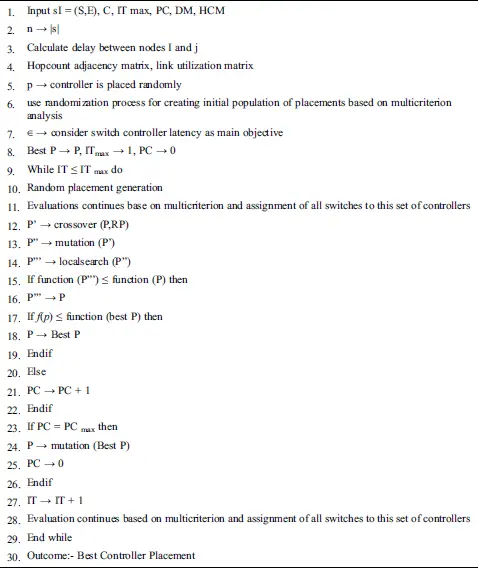5  Salp Swarm Algorithm

The SSA is a meta-heuristic algorithm that mathematically models the salp chain. The front salp of the chain is the leader and the rest of the salps behind the leader are followers. The leader leads the swarm for finding the food source, followed by the followers. Each salp position is an s-dimensional search space, where s is the number of variables. The salp position is stored in a 2D matrix called m, with food source F in the search space as the salp’s destination .

The update of the leader salp occurs based on the following equation.where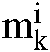is the leader salp position in kth dimension, and Fk is the food source position in kth dimension. uk,lk are the upper and lower boundaries of k-th dimension. c1, c2, c3 are random numbers used as model coefficients. The coefficient c1 is given byi = current iteration, I = maximum no of iteration. c2 and c3 are random numbers uniformly distributed in interval [0,1].

Follower’s position is updated based on newton’s law of motion which indicates of position of ith follower salp in kth dimension (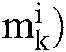and n is the total number of salps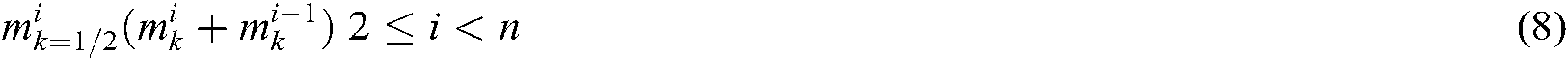6  Novel Chaotic Salp Swarm Algorithm

Meta-heuristic algorithms  use random parameters employing Gaussian or uniform distribution with recession in convergence. This chaos theory can be overcome by replacing random numbers with chaotic variables. The salp position is updated using c1 and c2 coefficients. c3 represents movement toward +ve or –ve infinity. c2 can be adjusted with chaotic map value at current iteration as follows [22,23]: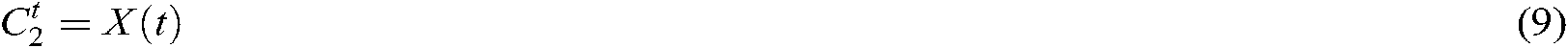Replace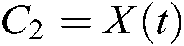Logistic chaotic map optimizer is used for updating coefficient c2x(t) = chaotic map value at t-th iteration and initial value x(0) is 0.7

7  Novel Chaotic Salp Swarm Algorithm (NCSSA) for Switch Controller Allocation

In this algorithm, the salp at the food source is the required optimized solution. The optimum number of controllers is assigned based on the multiple criteria advanced genetic algorithm. Now the optimum allocation of switches to controllers, based on NCSSA, is initialized based on the following algorithm :

Algorithm 2: NCSSA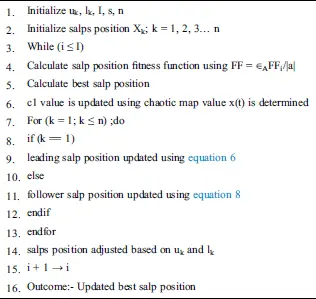8  Performance Evaluation

The performance of the NCSSA was evaluated through a simulation environment running on MATLAB. We selected 12 topologies from the Internet Topology Zoo and divided them into two categories, one with fewer switches and one with more switches. Tab. 1 shows the selected topologies. Tab. 2 shows the selected topologies in the two categories. The simulation parameters are listed in Tab. 3.

Table 1: Category1-TopologyTable 2: Category2-TopologyTable 3: Simulation Parameters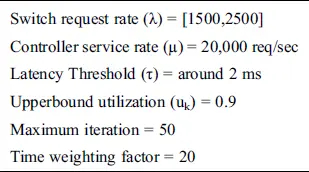The proposed algorithm was implemented for maximum iterations for each selected topology. Two different scenarios were considered based on the different values of latency threshold and upper boundary utilization. Tab. 4 shows the parameters used in two scenarios in four cases.

Table 4: Parameters for two scenariosFig. 3 shows the impact of the latency threshold on the two sets of selected topologies. An increase in the latency threshold allows the controller to provide response for more switches without affecting the required QoS. Therefore, with a higher latency threshold value, fewer controllers are needed to meet network requirements.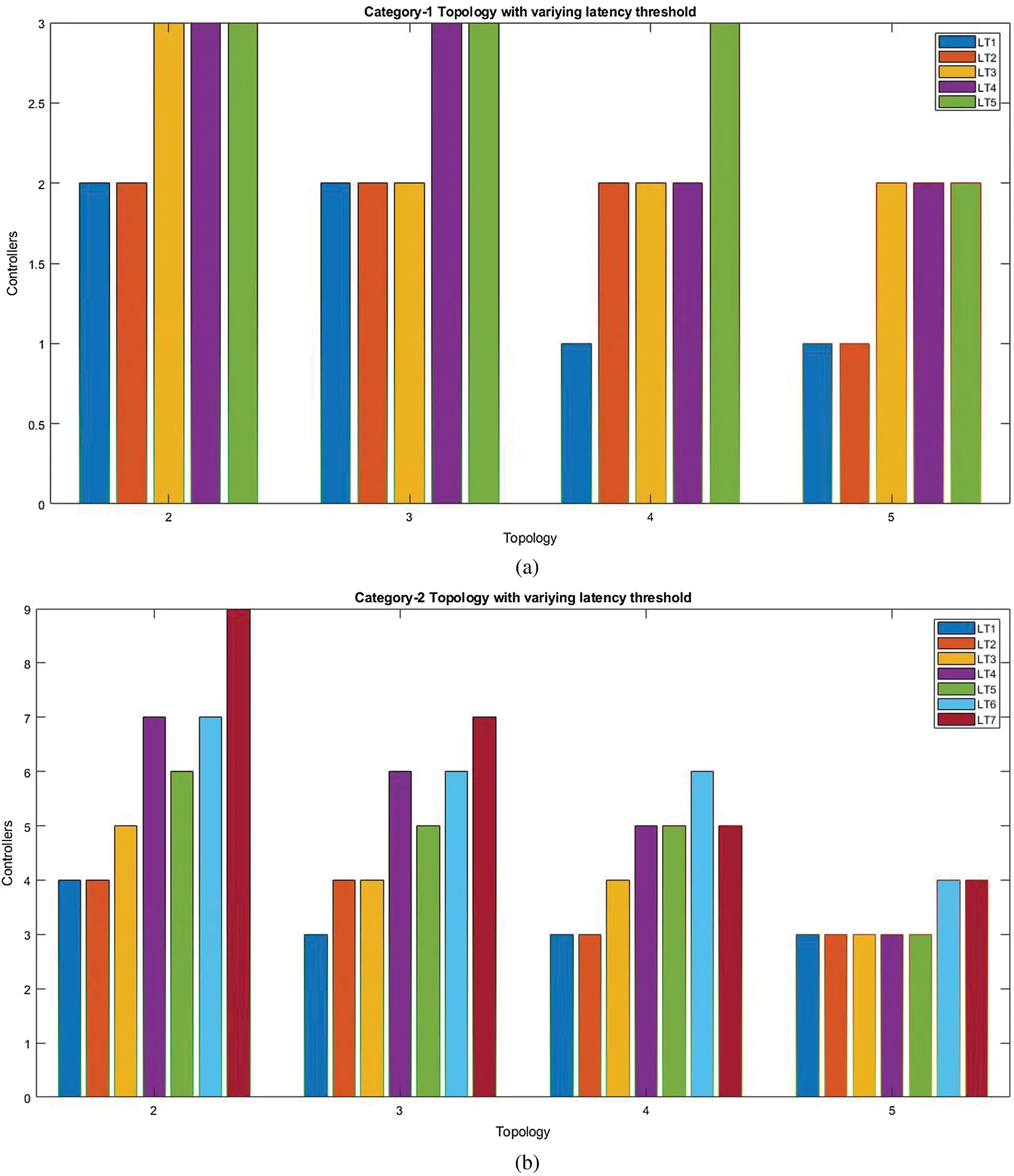Figure 3: Scenario 1 simulation results (a) category-1topologies (b) category-2 topologiesFigure 4: Scenario 2 simulation results (a) category-1topologies (b) category-2 topologies

Fig. 4 shows the impact of the utilization index on the two sets of selected topologies. The higher upper boundary utilization index (commonly used uk = 0.9 indicates 90% utilization) allows the controller to service more requests, so that fewer controllers need to be deployed.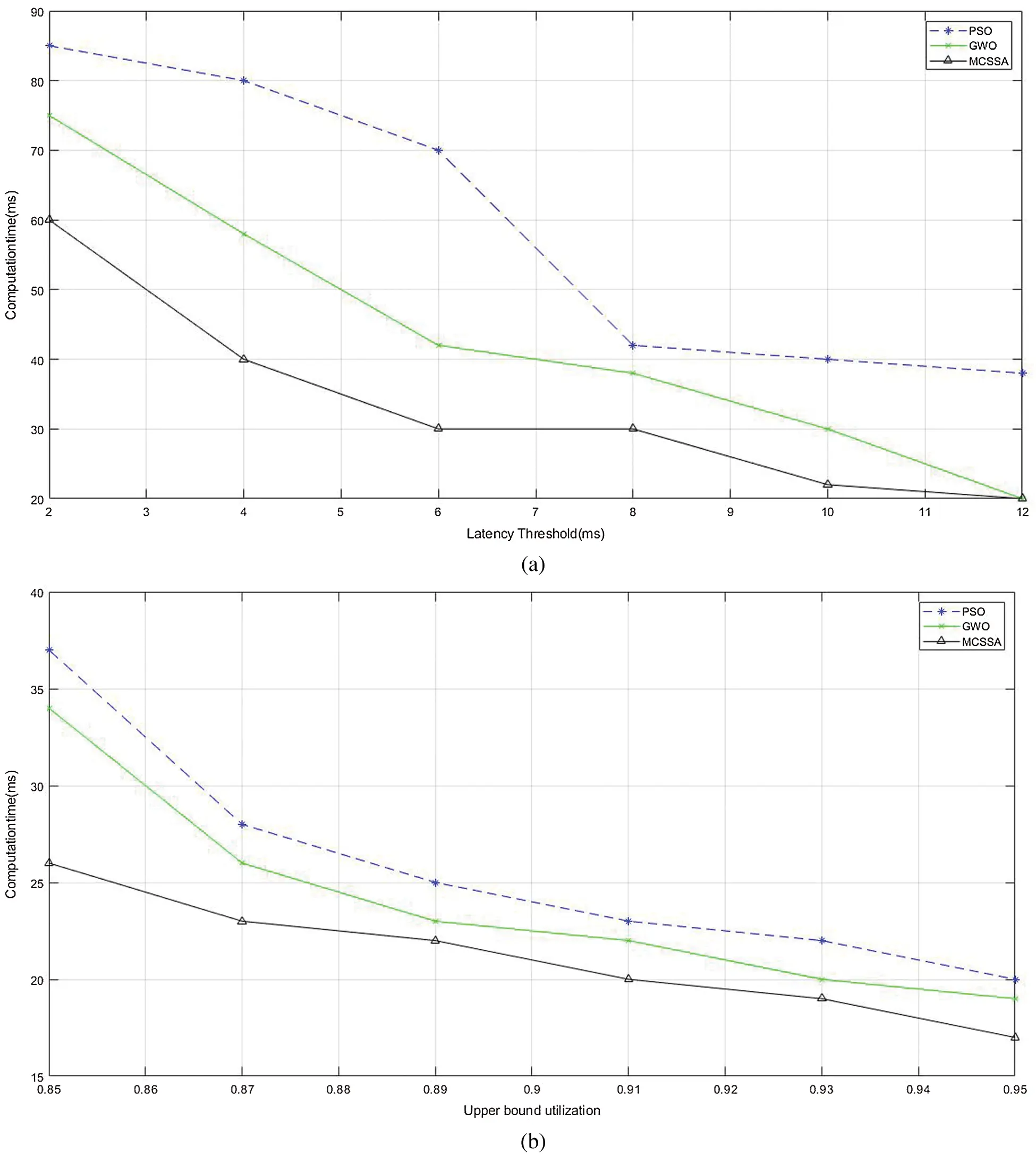Figure 5: Computation time with variation of (a) latency threshold (b) upper bound utilization

Fig. 5 shows a comparison of the computation times of NCSSA, the gray wolf optimization (GWO) algorithm and PSO. Computation time is an important metric in the consideration of faster execution, and achieving the required QOS for the different values of the latency threshold and the utilization index.

9  Conclusion

In this article, two algorithms are considered in a simulation environment: A multi-criterion advanced genetic algorithm for solving the problem of controller placement, based on criteria such as switch controller latency, hopcount, and link utilization, and a novel meta-heuristic chaotic salp swarm algorithm for switch controller allocation. The performance of the proposed algorithms are evaluated for 12 topologies, based on different metrics. The results confirmed that the algorithms optimized controller allocation and had faster computation times when compared with other algorithms.

Funding Statement: The author(s) received no specific funding for this study.

Conflicts of Interest: The authors declare that they have no conflicts of interest to report regarding the present study.

## References

1. J. H. Cox, J. Chung, S. Donovan, J. Ivey, R. J. Clark et al. (2017). , “Advancing software-defined networks: A survey,” IEEE Access, vol. 5, pp. 25487–25526.
2. G. Nencioni, R. G. Garroppo, A. J. Gonzalez, B. E. Helvik and G. Procissi. (2018). “Orchestration and control in software-defined 5G networks: research challenges,” Wireless Communications and Mobile Computing, vol. 2018, no. 2, pp. 1–18.
3. A. K. Singh and S. Srivastava. (2019). “A survey and classification of controller placement problem in SDN,” International Journal of Network Management, vol. 7, pp. 1–25.
4. C. Guo, C. Gong, J. Guo, H. Xu and L. Zhang. (2019). “TCQG—software-defined transmission control scheme in 5G networks from queuing game perspective,” Sensors, vol. 19, no. 19, pp. 4170–4187.
5. S. K. Tayyaba and M. A. Shah. (2019). “Resource allocation in SDN based 5G cellular networks,” Peer-to-Peer Networking and Applications, vol. 12, no. 2, pp. 514–538.
6. A. A. Ateya, A. Muthanna, A. Vybornova, A. D. Algarni, A. Abuarqoub et al. (2019). , “Chaotic salp swarm algorithm for SDN multi-controller networks,” Engineering Science and Technology, an International Journal, vol. 22, no. 4, pp. 1001–1012.
7. A. A. A. Ari, A. Gueroui, C. Titouna, O. Thiare and Z. Aliouat. (2019). “Resource allocation scheme for 5G C-RAN: A swarm intelligence based approach,” Computer Networks, vol. 165, 10695
8. A. A. Barakabitze, A. Ahmad, R. Mijumbi and A. Hines. (2020). “5G network slicing using SDN and NFV: A survey of taxonomy, architectures and future challenges,” Computer Networks, vol. 167, pp. 106984.
9. A. Sallahi and M. St-Hilaire. (2017). “Expansion model for the controller placement problem in software defined networks,” IEEE Communications Letters, vol. 21, no. 2, pp. 274–277.
10. H. K. Rath, V. Revoori, S. M. Nadaf and A. Simha. (2014). “Optimal controller placement in software defined networks (SDN) using a non-zero-sum game,” in Proc. IEEE International Symposium on a World of Wireless, Mobile and Multimedia Networks 2014, Sydney, NSW, pp. 1–6.
11. C. Gao, H. Wang, F. Zhu, L. Zhai and S. Yi. (2015). “A particle swarm optimization algorithm for controller placement problem in software defined network,” in Proc. Int. Conf. on Algorithms and Architectures for Parallel Processing, Cham: Springer, 9530, pp. 44–54.
12. M. F. Bari, A. R. Roy, S. R. Chowdhury, Q. Zhang and F. M. (2013). “Zhani etal, Dynamic controller provisioning in software defined networks,” in Proc of the 9th Int. Conf. on Network and Service Management (CNSM 2013pp. 18–25.
13. A. Jalili, M. Keshtgari, R. Akbari and R. Javidan. (2019). “Multi criteria analysis of controller placement problem in software defined networks,” Computer Communications, vol. 133, pp. 115–128.
14. N. Mouawad, R. Naja and S. Tohme. (2018). “Optimal and dynamic SDN controller placement,” in Proc. 2018 Int. Conf. on Computer and Applications (ICCABeirut, pp. 1–9.
15. G. Wang, Y. Zhao, J. Huang and Y. Wu. (2018). “An effective approach to controller placement in software defined wide area networks,” IEEE Transactions on Network and Service Management, vol. 15, no. 1, pp. 344–355.
16. T. Wang, F. Liu and H. Xu. (2017). “An efficient online algorithm for dynamic SDN controller assignment in data center networks,” IEEE/ACM Transactions on Networking, vol. 25, no. 5, pp. 2788–2801.
17. J. Liu, Y. Shi, L. Zhao, Y. Cao, W. Sun and N. Kato. (2018). “Joint placement of controllers and gateways in SDN-enabled 5G-satellite integrated network,” IEEE Journal on Selected Areas in Communications, vol. 36, no. 2, pp. 221–232.
18. H. K. Rath, V. Revoori, S. M. Nadaf and A. Simha. (2014). “Optimal controller placement in software defined networks (SDN) using a non-zero-sum game,” in Proc. IEEE Int. Sym. on a World of Wireless, Mobile and Multimedia Networks 2014, Sydney, NSW, pp. 1–6.
19. A. Farshin and S. Sharifian. (2017). “A chaotic grey wolf controller allocator for software defined mobile network (SDMN) for 5th generation of cloud-based cellular systems (5G),” Computer Communications, vol. 108, pp. 94–109.
20. R. A. Ibrahim, A. A. Ewees, D. Oliva, M. A. Elaziz and S. Lu. (2019). “Improved salp swarm algorithm based on particle swarm optimization for feature selection,” Journal of Ambient Intelligence and Humanized Computing, vol. 10, no. 8, pp. 3155–3169.
21. A. Farshin and S. Sharifian. (2017). “MAP-SDN: A metaheuristic assignment and provisioning SDN framework for cloud datacenters,” Journal of Supercomputing, vol. 73, no. 9, pp. 4112–4136.
22. G. I. Sayed, G. Khoriba and M. H. Haggag. (2018). “A novel chaotic salp swarm algorithm for global optimization and feature selection,” Applied Intelligence, vol. 48, no. 10, pp. 3462–3481.
23. M. A. Maitah, O. O. Semenova, A. O. Semenov, P. I. Kulakov and V. Y. Kucheruk. (2018). “A hybrid approach to call admission control in 5G networks,” Advances in Fuzzy Systems, vol. 20, no. 4, pp. 1–7.
24. A. K. Tran, M. J. Piran and C. Pham. (2019). “SDN controller placement in IoT networks: An optimized submodularity-based approach,” Sensors, vol. 19, no. 24, pp. 5474–5489.This work is licensed under a Creative Commons Attribution 4.0 International License, which permits unrestricted use, distribution, and reproduction in any medium, provided the original work is properly cited.